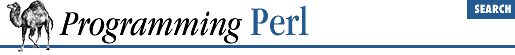#Chapter 3Functions### 3.2.182 unpack

`unpack `TEMPLATE`, `EXPR``

This function does the reverse of pack: it takes a string (`EXPR`) representing a data structure and expands it out into a list value, returning the list value. (In a scalar context, it can be used to unpack a single value.) The `TEMPLATE` has much the same format as in the pack function - it specifies the order and type of the values to be unpacked. (See pack for a more detailed description of `TEMPLATE`.)

Here's a subroutine that does (some of) substr, only slower:

```sub substr {
my(\$what, \$where, \$howmuch) = @_;
if (\$where < 0) {
\$where = -\$where;
return unpack "\@* X\$where a\$howmuch", \$what;
}
else {
return unpack "x\$where a\$howmuch", \$what;
}
}```

and then there's:

`sub signed_ord { unpack "c", shift }`

Here's a complete uudecode program:

```#!/usr/bin/perl
\$_ = <> until (\$mode,\$file) = /^begin\s*(\d*)\s*(\S*)/;
open(OUT,"> \$file") if \$file ne "";
while (<>) {
last if /^end/;
next if /[a-z]/;
next unless int((((ord() - 32) & 077) + 2) / 3) ==
int(length() / 4);
print OUT unpack "u", \$_;
}
chmod oct \$mode, \$file;```

In addition, you may prefix a field with `%``number` to indicate that you want it to return a `number`-bit checksum of the items instead of the items themselves. Default is a 16-bit checksum. For example, the following computes the same number as the System V sum program:

```undef \$/;
\$checksum = unpack ("%32C*", <>) % 32767;```

The following efficiently counts the number of set bits in a bit vector:

`\$setbits = unpack "%32b*", \$selectmask;`

Here's a simple MIME decoder:

```while (<>) {
tr#A-Za-z0-9+/##cd;                   # remove non-base64 chars
tr#A-Za-z0-9+/# -_#;                  # convert to uuencoded format
\$len = pack("c", 32 + 0.75*length);   # compute length byte
print unpack("u", \$len . \$_);         # uudecode and print
}```